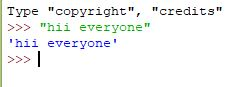GFG App
Open AppBrowser
Continue

# Taking input from console in Python

What is Console in Python? Console (also called Shell) is basically a command line interpreter that takes input from the user i.e one command at a time and interprets it. If it is error free then it runs the command and gives required output otherwise shows the error message. A Python Console looks like this.Here we write a command and to execute the command just press enter key and your command will be interpreted. For coding in Python, you must know the basics of the console used in Python. The primary prompt of the python console is the three greater than symbols

`>>>`

You are free to write the next command on the shell only when these prompts have appeared after executing the first command. The Python Console accepts commands in Python that you write after the prompt.Accepting Input from Console User enters the values in the Console and that value is then used in the program as it was required. To take input from the user we make use of a built-in function input()

## Python

 `# input` `input1 ``=` `input``()`   `# output` `print``(input1)`

We can also typecast this input to integer, float, or string by specifying the input() function inside the type.

1. Typecasting the input to Integer: There might be conditions when you might require integer input from the user/Console, the following code takes two input(integer/float) from the console and typecasts them to an integer then prints the sum.

## Python

 `# input` `num1 ``=` `int``(``input``())` `num2 ``=` `int``(``input``())`   `# printing the sum in integer` `print``(num1 ``+` `num2)`

2. Typecasting the input to Float: To convert the input to float the following code will work out.

## Python

 `# input` `num1 ``=` `float``(``input``())` `num2 ``=` `float``(``input``())`   `# printing the sum in float` `print``(num1 ``+` `num2)`

3. Typecasting the input to String: All kinds of input can be converted to string type whether they are float or integer. We make use of keyword str for typecasting.

we can also take input string by just writing input() function by default it makes the input string

## Python

 `# input` `string ``=` `str``(``input``())`   `# output` `print``(string)`   `# Or by default` `string_default ``=` `input``()`   `# output` `print``(string_default)`

My Personal Notes arrow_drop_up